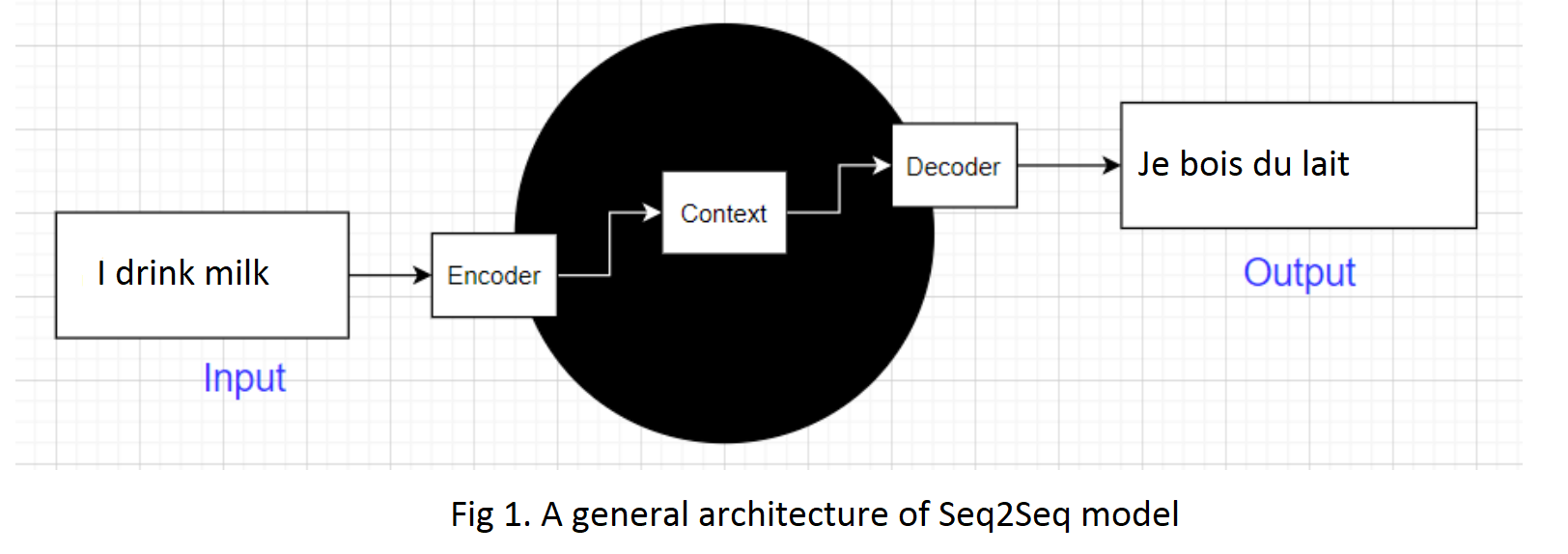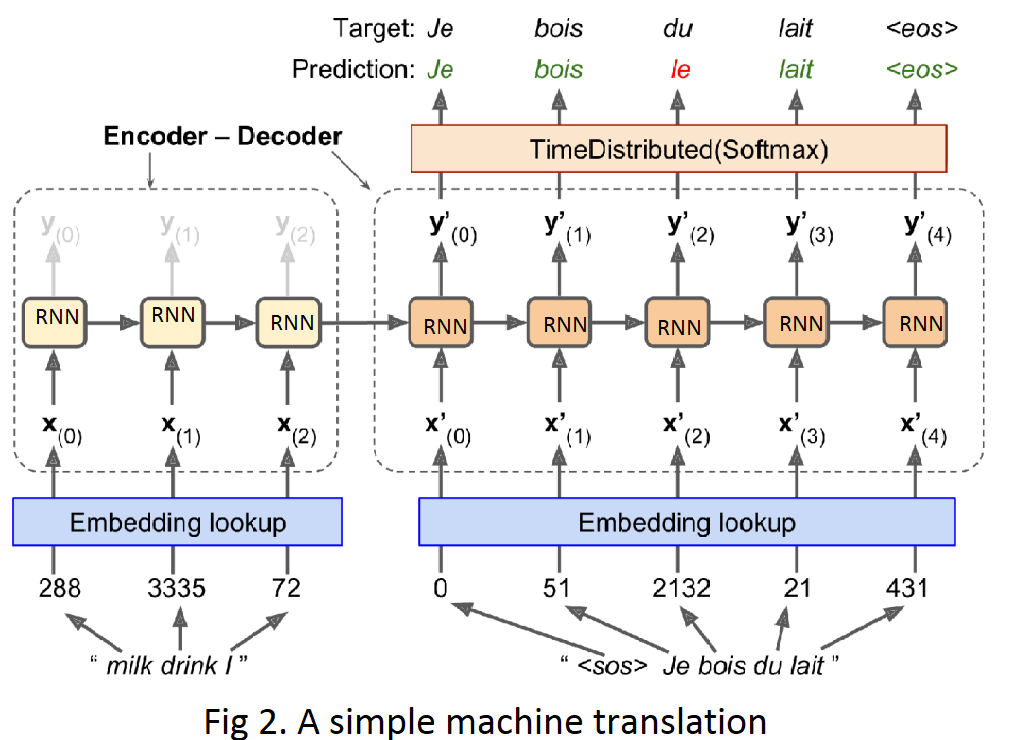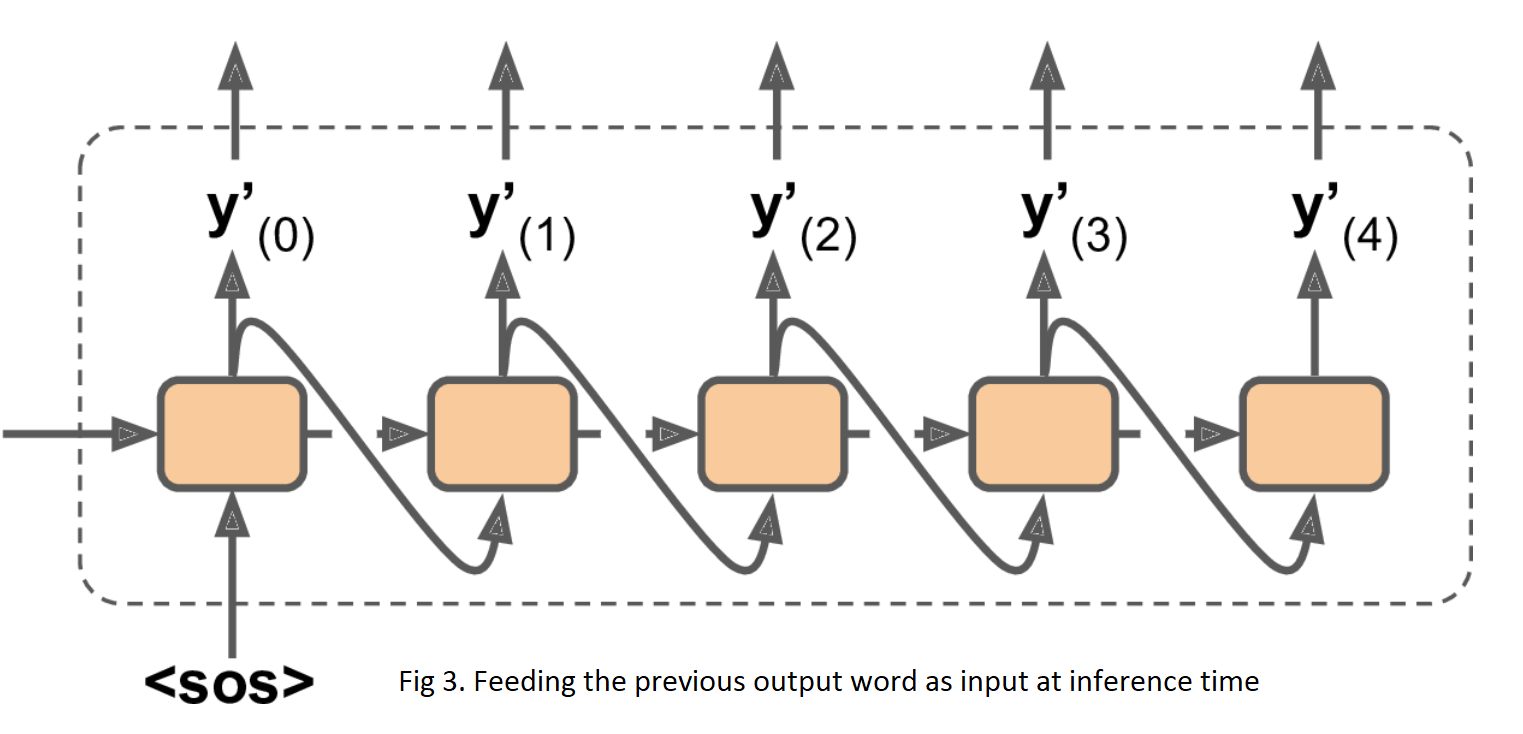×

Search anything:

# Seq2seq: Encoder-Decoder Sequence to Sequence Model Explanation

#### Machine Learning (ML) Deep LearningIn Deep learning, we all know that Recurrent Neuron Network solves time series data. Sequence to Sequence (or Seq2Seq for short) is a kind of model that was born to solve "Many to many" problem. Seq2Seq has many applications,perhaps the most common one is Machine Translation. In this article, we will explore its architecture and how to apply it with code.Before starting , if you haven't been familiar with LSTM and GRU , please read these posts.

# Architecture and mechanism

Our model's input and output are both sequence. In this article, input is a sentence in English and output is a sentence in French.Model's architecture has 2 components: encoder and decoder. When encoder is fed an input, decoder outputs a sentence.Note that this output is used as input of encoder in the next step. In other words, output of this step is utilized as a part of input in the next step.
For the very first word,it is given the start-of-sequence (SOS) token. The decoder is expected to end the sentence with an end-of-sequence (EOS) token.
Note that the input sentences are reversed before they are fed to the encoder.For example ," I drink milk " is reversed to "milk drink I".This ensures that the beginning of the English sentence will be fed last to the encoder, which is useful because that’s generally the first thing that the decoder needs to translate.
Now we come to details. See the figure 2 and I'll explain it :### Embedding lookup

Before feed the encoder, we need to preprocessing our input which is text.All punctuation is removed, and then words are converted to lowercase, split by spaces, and finally indexed by frequency.The 0,1,2 are special: they represent the padding token,the start-of-sequence and unknown words,respectively.
First, each word is initially represented by its ID(e.g. 288 for the word "milk",3335 for the "drink").Then, the Embedding layer returns the word embedding which is a vector with predefined number of dimensions. These word embeddings are actually input of encoder.

### Encoder

An encoder is a stack of recurrent units which can be replaced by LSTM of GRU cells for better performance.
At each step, the recurrent unit takes in one embeded word corresponding to input x_t and returns hidden state h_t.Then the next recurrent unit takes in h_t and x_t+1 as its input and returns h_t+1.This cycle repeats again and again with other sentences.

### Context vector

Context vector or Encoder vector is the final hidden state of encoder part.It encapsulates all the information of input sentence in order to make output more accurate. Context vector is also the input of decoder.

### Decoder

A decoder shares the similar structure and operation with encoder, except one difference: each recurrent unit returns both output y_t and hidden state h_t, they are the same in almost cases .Each hidden state is computed by this formula :Each output y_t can be consider as a score for one word of output vocabulary(i.e French). They are fed to Softmax Layer to calculate the probabilities.For example, at the first step the word “Je” may have a probability of 20%, “Tu” may have a probability of 1%, and so on. The word with the highest probability is output.
Note that at inference time (after training), you will not have the target sentence to feed to the decoder. Instead, simply feed the decoder the word that it output at the previous step, as shown in figure 3.### Timedistributed softmax layer

To turn the model into a sequence-to-sequence model, we must set return_sequences=True in all recurrent layers (even the last one), and we must apply the output Dense layer at every time step. Keras offers a TimeDistributed layer for this very purpose: it wraps any layer (e.g., a Dense layer) and applies it at every time step of its input sequence. It does this efficiently, by reshaping the inputs so that each time step is treated as a separate instance (i.e., it reshapes the inputs from [batch size, time steps,input dimensions] to [batch size × time steps, input dimensions] then it runs the Dense layer, and finally it reshapes the outputs back to sequences (i.e., it reshapes the outputs from [batch size × time steps, output dimensions] to [batch size, time steps, output dimensions].

# Application

Now it's time for coding.First, we build a basic encoder-decoder model like the one in figure 2.
First, we declare the embed_size and vocab_size so that we can use them to define Embedding layer.

``````embed_size = 10
vocab_size = 100
``````

Create input layers for encoder and decoder, note that we set shape=[None] here and dtype = np.int32 because we only use integers to represent index of words.Don't forget that in machine translation task,in above case, encoder_inputs are sentences in English and decoder_inputs are sentences in French.

``````encoder_inputs = keras.layers.Input(shape=[None], dtype=np.int32)
decoder_inputs = keras.layers.Input(shape=[None], dtype=np.int32)
sequence_lengths = keras.layers.Input(shape=[], dtype=np.int32)
embeddings = keras.layers.Embedding(vocab_size, embed_size)
``````

Next, we feed embedding layers encoder_inputs and decoder_inputs. They are transformed into vectors that our RNN cells could understand.

``````encoder_embeddings = embeddings(encoder_inputs)
decoder_embeddings = embeddings(decoder_inputs)
``````

In this cell, we define a LSTM neuron network with 512 units. we set return_state=True when creating the LSTM layer so that we can get its final hidden state and pass it to the decoder. Since we are using an LSTM cell, it actually returns two hidden states (short term and long term).
Another option is using GRU cell,but in this case, you need to set return_sequences=True

``````encoder = keras.layers.LSTM(512, return_state=True)
encoder_outputs, state_h, state_c = encoder(encoder_embeddings)
encoder_state = [state_h, state_c]
``````

Finally, we define our decoder part:

``````import tensorflow_addons as tfa
sampler = tfa.seq2seq.sampler.TrainingSampler()
decoder_cell = keras.layers.LSTMCell(512)
output_layer = keras.layers.Dense(vocab_size)
decoder = tfa.seq2seq.basic_decoder.BasicDecoder(decoder_cell,sampler,output_layer=output_layer)
final_outputs, final_state, final_sequence_lengths = decoder(
decoder_embeddings, initial_state=encoder_state,
sequence_length=sequence_lengths)
Y_proba = tf.nn.softmax(final_outputs.rnn_output)
model = keras.Model(inputs=[encoder_inputs,decoder_inputs,sequence_lengths],outputs=[Y_proba])
``````

The TrainingSampler is one of several samplers available in TensorFlow Addons: their role is to tell the decoder at each step what it should pretend the previous output was. During inference, this should be the embedding of the token that was actually output. During training, it should be the embedding of the previous target token: this is why we used the TrainingSampler.
You can also replace tf.nn.softmax with TimeDistributed(Dense(...)),set activation='softmax' as we discussed before .They are the same.

Compile model:

``````model.compile(loss="sparse_categorical_crossentropy", optimizer="adam")
``````

Now we come to the next step, create pseudo-data.If you want to work with real data( English-French translation),you can find the full code with very clear explaination in my colab .

``````X = np.random.randint(100, size=10*1000).reshape(1000, 10)
Y = np.random.randint(100, size=15*1000).reshape(1000, 15)
X_decoder = np.c_[np.zeros((1000, 1)), Y[:, :-1]]
seq_lengths = np.full(, 15)

history = model.fit([X, X_decoder, seq_lengths], Y, epochs=2)
``````

After training progress, we get the result:

``````Epoch 1/2
32/32 [==============================] - 6s 6ms/sample - loss: 4.6053
Epoch 2/2
32/32 [==============================] - 3s 3ms/sample - loss: 4.6031
``````

# Discussion

In this part, we discuss about some pros and cons of our model.

• Pros:
easy to apply due to its simplicity.
could map an input sentence with a target sentence with different length.
• Cons:
It takes in a sentence and transform it into a sentence in another language through
only one vector (context vector). If input are long sentences, it's difficult for decoder to generate sentences accurately through only context vector because we are trying to cram an entire sentence into encoder regardless how many words compose that sentence.
Moreover,RNN models loses more or less information of some first nodes, so the context vector can not learn all the information of encoder.This classic model learns short sentences very well, but it performs badly when trying to capture long sentences.Once again, this drawback comes from the limited short-term memory of RNNs.

These problem leads us to another model: Attention.This SOTA model shortens the path from input words to their target words.For example, at the time step where the decoder needs to output the word “lait,” it will focus its attention on the word “milk.” This means that the path from an input word to its translation is now much shorter, so the short-term memory limitations of RNNs have much less impact.
We will discuss about it in other article at OpenGenus.

#### Nguyen Quoc Trung

Student at Ha Noi University of Science and Technology, ML Developer Intern at OpenGenus | I like reading books, playing chess and sports and want create my own AI startup to change the world.

Seq2seq: Encoder-Decoder Sequence to Sequence Model Explanation